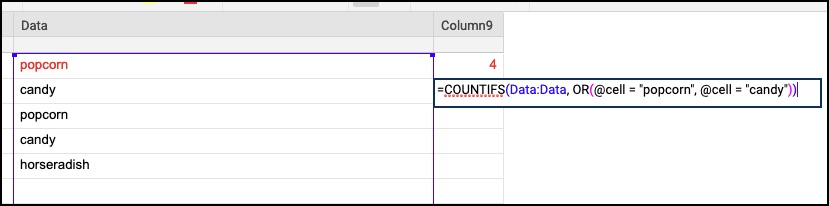# I can't figure out how to use the OR function in a Countifs formula

Hello,

I have been creating metrics on my data in smartsheet using the different formulas.  I have figured many out (with a lot of head banging and sleepless nights), but cannot figure out how to use the OR function.

works: =COUNTIFS({Week}, =\$[Date Range]\$3, {Month}, \$[Date Range]\$5, {Year}, \$[Date Range]\$8, {task status}, "PCC Case Submitted")

Does not work: =COUNTIFS({Week}, =\$[Date Range]\$3, {Month}, \$[Date Range]\$5, {Year}, \$[Date Range]\$8, OR({task status}, "PCC Case Submitted", "PCC Case Now Closed"))

What I am doing wrong with the or functionality?

• You would want to use @cell = for each of your criteria.

Try something like this:

=COUNTIFS({Week}, =\$[Date Range]\$3, {Month}, \$[Date Range]\$5, {Year}, \$[Date Range]\$8, {task status} OR(@cell =  "PCC Case Submitted", @cell = "PCC Case Now Closed"))

• I've attached a screenshot showing a simplified version of the formula.• Mike,

Forgot a comma in the posted formula after {task Status}.

=COUNTIFS({Week}, =\$[Date Range]\$3, {Month}, \$[Date Range]\$5, {Year}, \$[Date Range]\$8, {task status}, OR(@cell =  "PCC Case Submitted", @cell = "PCC Case Now Closed"))

.

.

Colleen,

Another part of the problem with your formula is that you are including your range within the OR function. You should only include the criteria (and Mike's correction of using @cell).

Original:

OR({task status}, "PCC Case Submitted", "PCC Case Now Closed")

Corrected:

{task status}, OR(@cell = "PCC Case Submitted", @cell = "PCC Case Now Closed")

• Thanks, Paul! Good catch.

•## Help Article Resources

Want to practice working with formulas directly in Smartsheet?

Check out the Formula Handbook template!